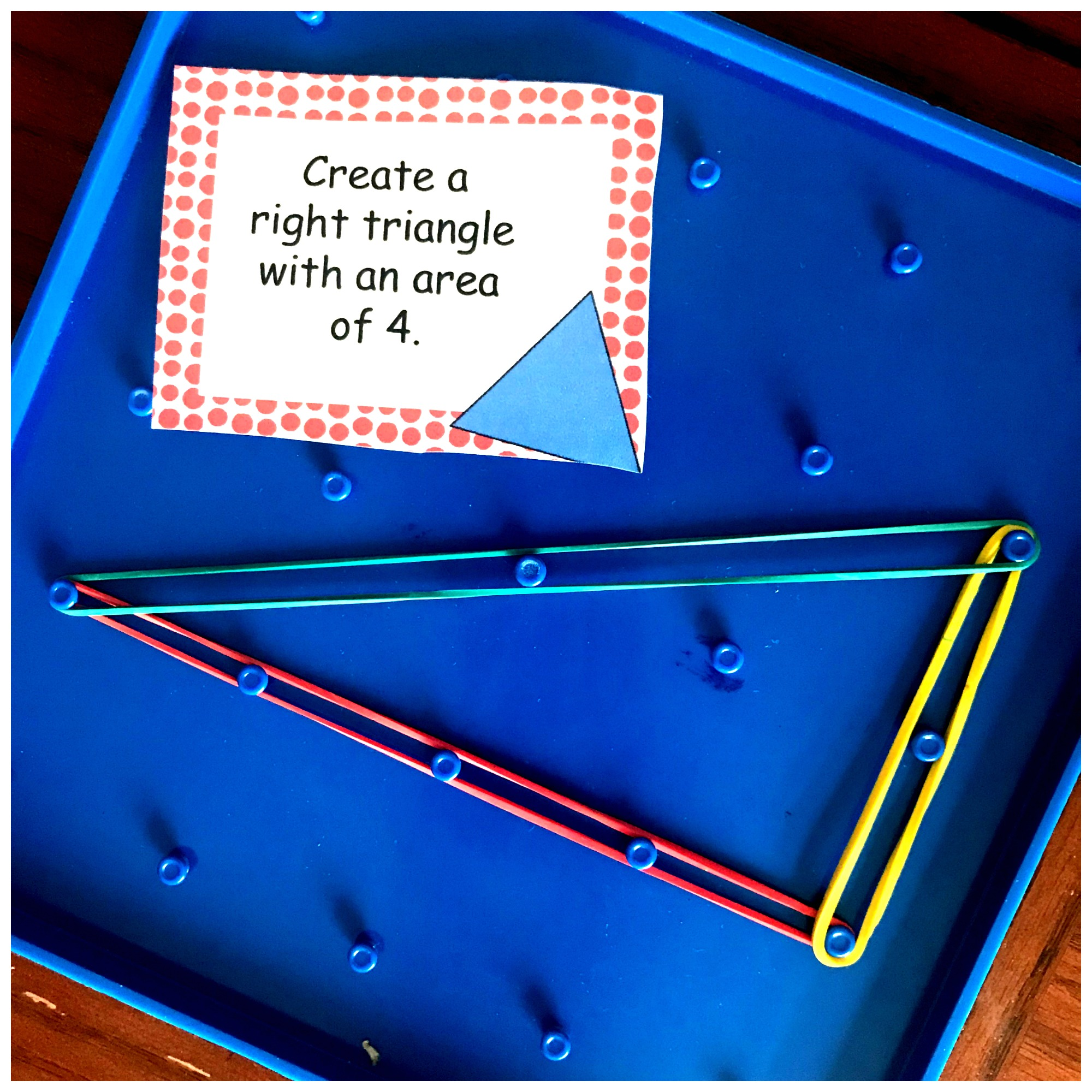Home » Math » FREE Clip Cards For Different Types Of Triangles

# FREE Clip Cards For Different Types Of Triangles

Your students will love these FREE clip cards for different types of triangles. Have them recognize and name scalene, isosceles, equilateral, right, obtuse and acute triangles.This week my son was introduced to triangles. Ok, he has known what a triangle is for a very long time. But this week he found out that triangles have different names.

Yep, it was our week to learn about isosceles, equilateral, and scalene triangles. But we can’t leave it at that, can we? We also had to focus on right triangles, acute triangles, and obtuse triangles.

Our math book had us study the triangles and figure out the rules for each triangle by observing their sides and angles.

Then we moved unto a simple game where he drew triangles and then figured out what they were.

Today, it is time for a simple review of the different types of triangles.

## Prep-Work

Grab some scissors and this activity will be ready in no time.

1. First, print off the clip cards on card stock paper and print off the recording sheet on normal paper.
2. Next, cut out the clip cards and laminate for multiple uses.
3. Finally, gather up some clothes pens and pencils and you are ready to go.

## Different Types Of Triangles

Just in case you haven’t talked about triangles in a while, and need a little refresher course…..here is what you and your kiddos can be on the lookout for when naming triangles.

#### Equilateral Triangle

This triangle is an easy one. Just as its name says, we are looking for a triangle with all sides that are equal.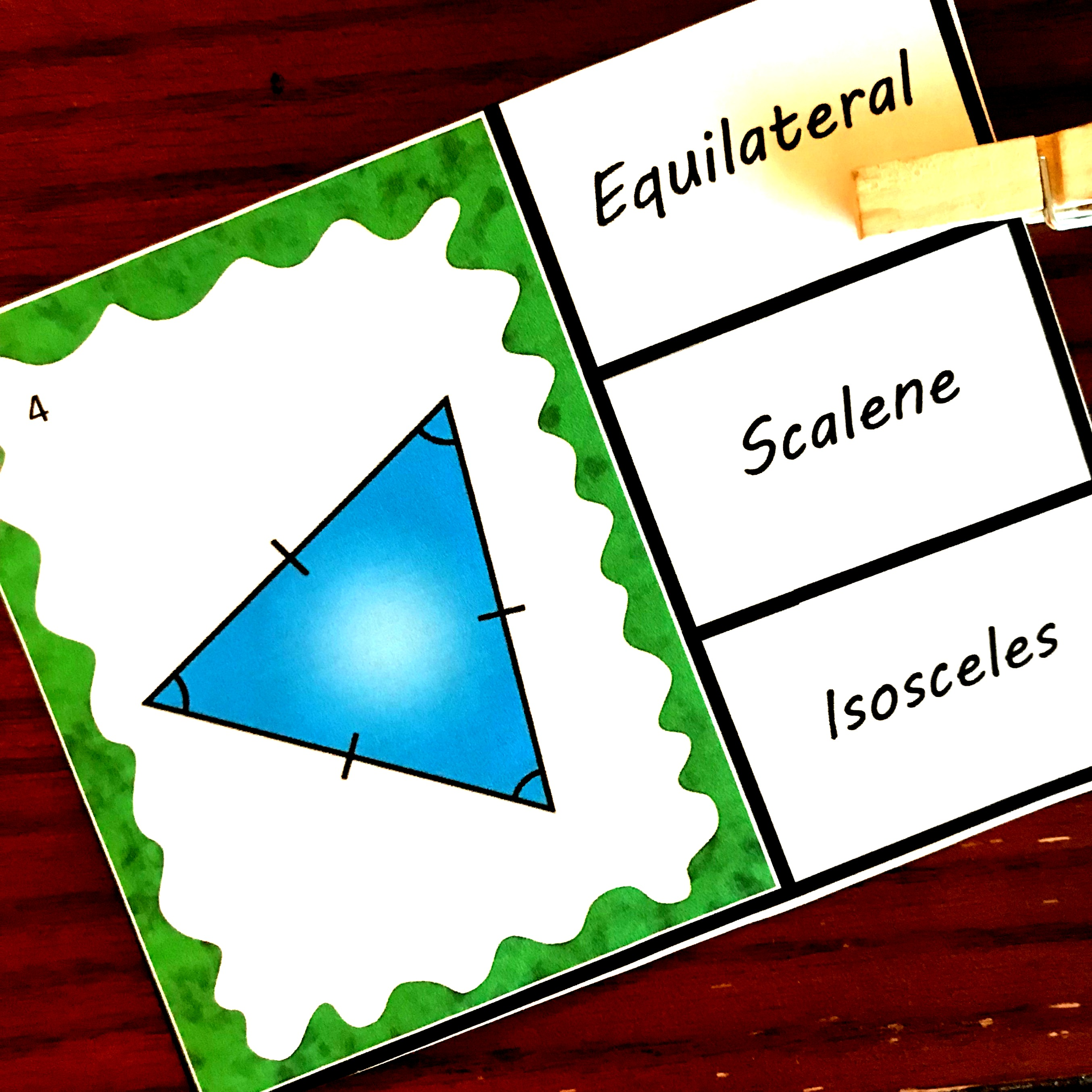#### Isosceles Triangle

With an isosceles triangle, we move from all sides being equal length, to just two sides being equal length.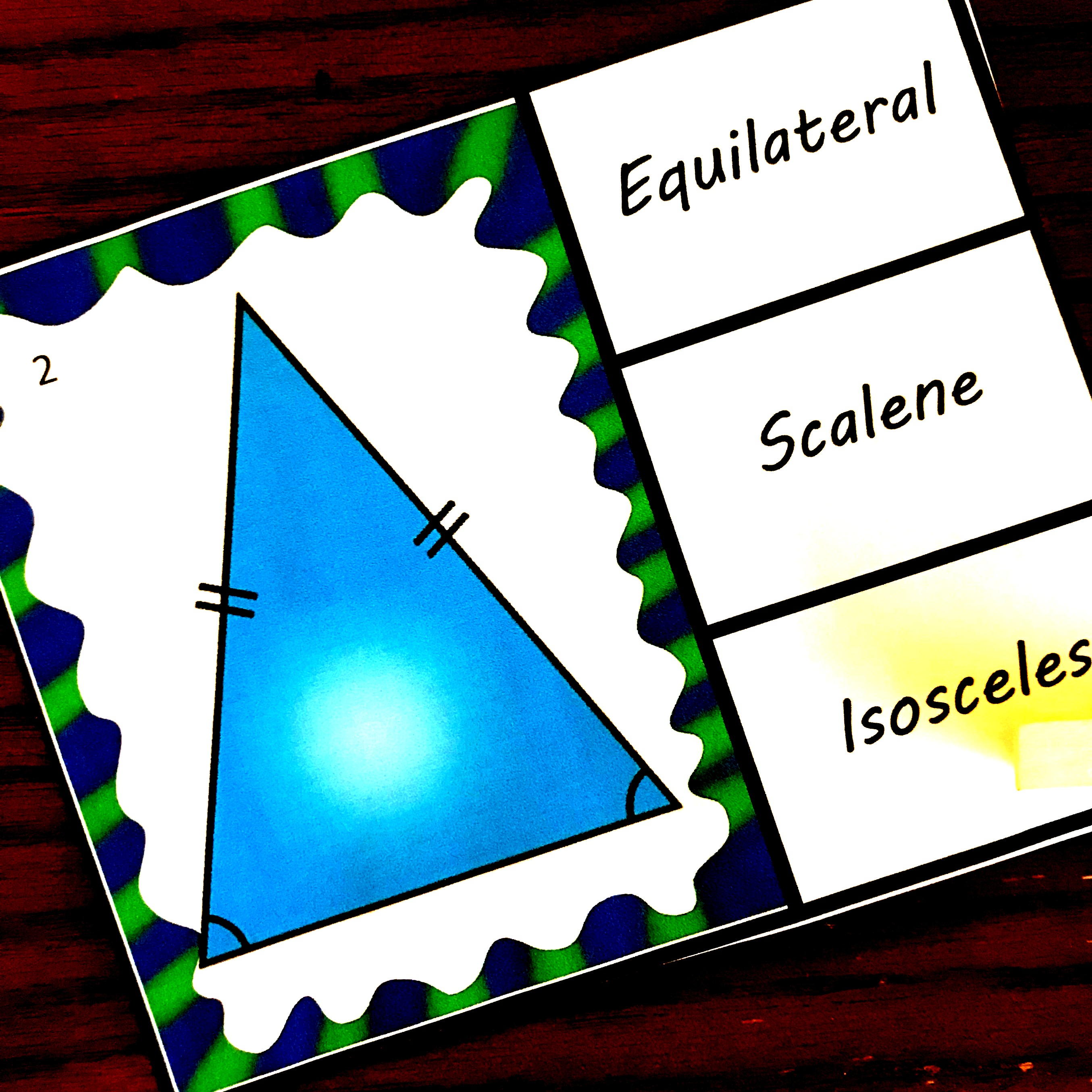#### Scalene Triangle

Can you guess which this one is? Yep, it does not have any equal sides. All sides are of different lengths.#### Right Triangle

Now we move from looking at sides to studying angles. In this triangle, one angle must be a right angle. This means that one angle is 90 degrees or you can say looks like an L.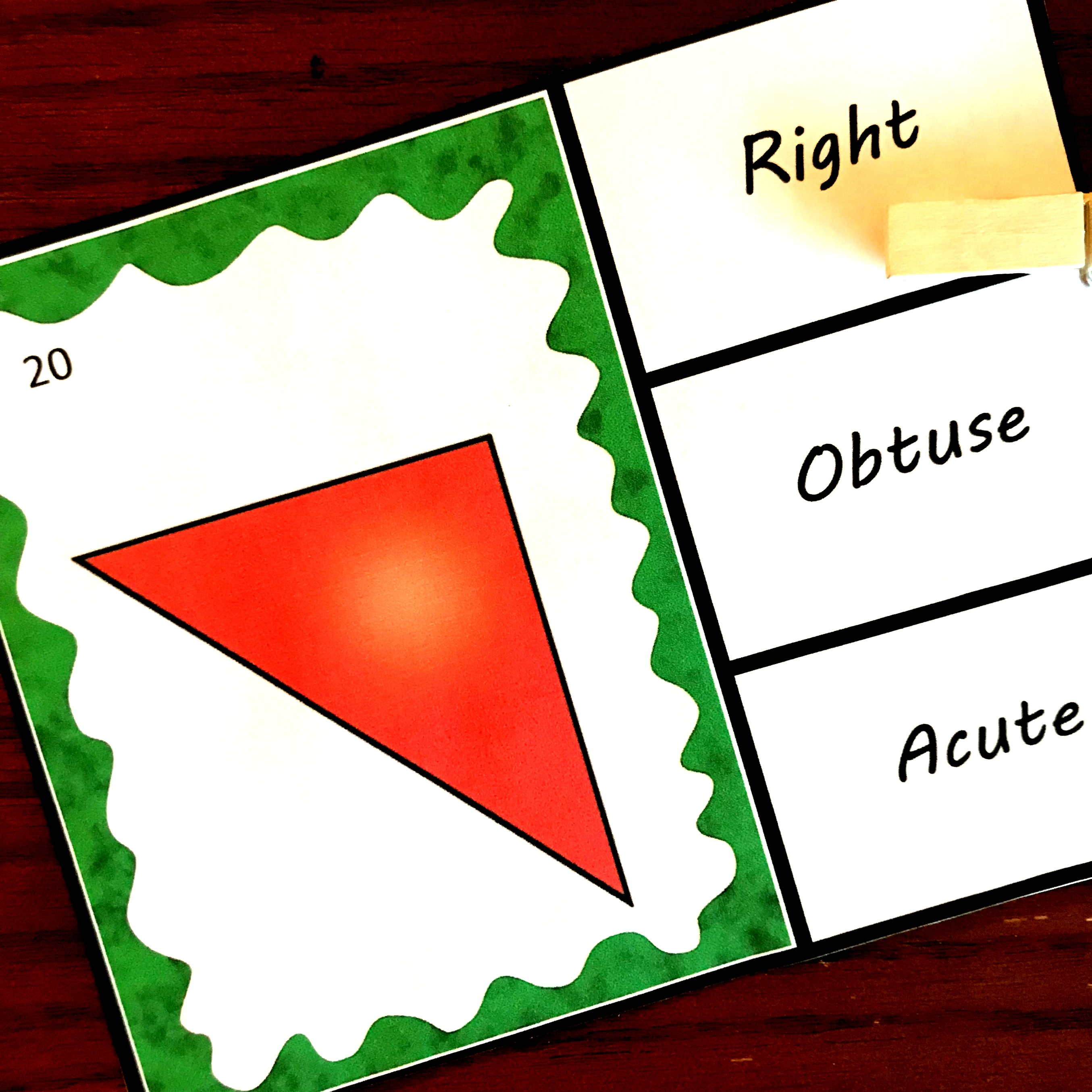#### Acute Triangle

An acute triangle is a pretty easy one. All angles are acute…or less than 90 degrees.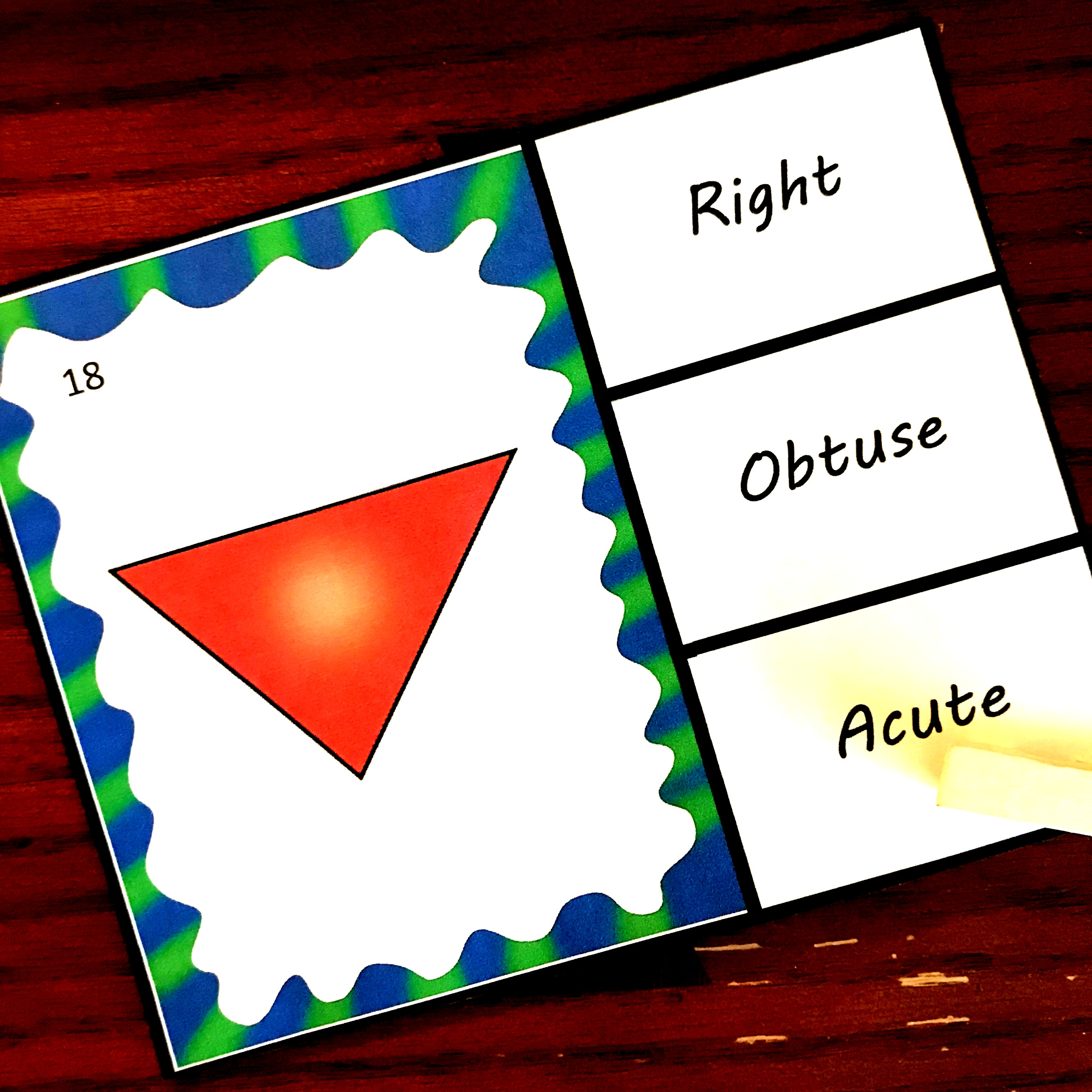#### Obtuse Triangle

And finally, we have the obtuse triangle. These triangles have one obtuse angle…or an angle that is over 90 degrees.

I hope your kiddos enjoy this simple review.

You’ve Got This

You may also like:

Finding the area of a triangle interactive notebook:Building triangles based on the area